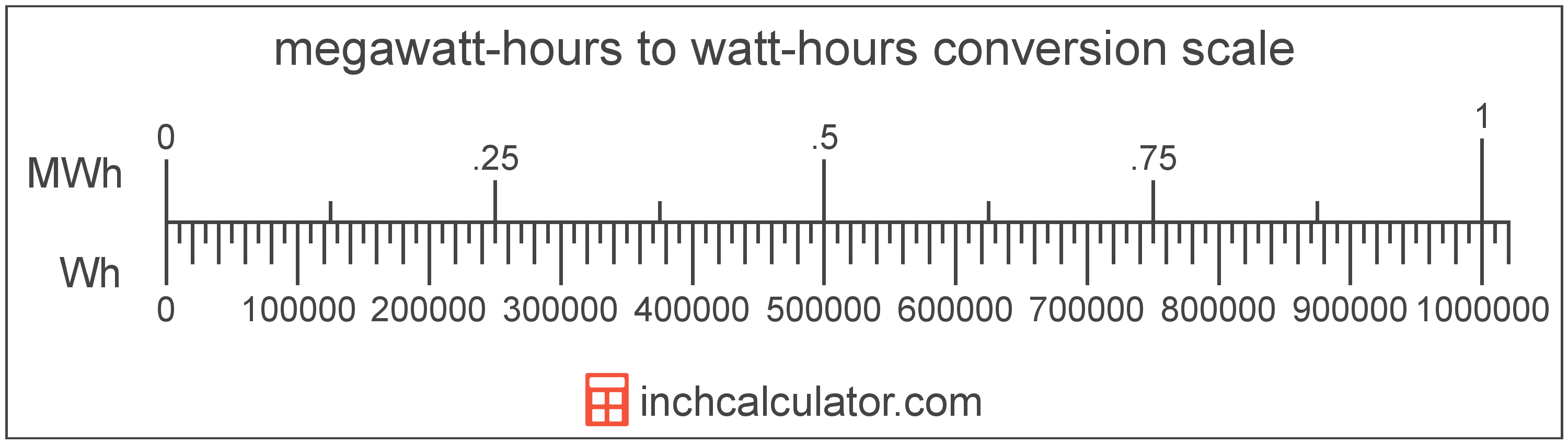# Megawatt-hours to Watt-hours Conversion

Enter the energy in megawatt-hours below to get the value converted to watt-hours.

Results in Watt-hours:1 MWh = 1,000,000 Wh

## How to Convert Megawatt-hours to Watt-hoursTo convert a megawatt-hour measurement to a watt-hour measurement, multiply the energy by the conversion ratio.

Since one megawatt-hour is equal to 1,000,000 watt-hours, you can use this simple formula to convert:

watt-hours = megawatt-hours × 1,000,000

The energy in watt-hours is equal to the megawatt-hours multiplied by 1,000,000.

For example, here's how to convert 5 megawatt-hours to watt-hours using the formula above.
5 MWh = (5 × 1,000,000) = 5,000,000 Wh

### How Many Watt-hours are in a Megawatt-hour?

There are 1,000,000 watt-hours in a megawatt-hour, which is why we use this value in the formula above.

1 MWh = 1,000,000 Wh

## Megawatt-hours

A megawatt-hour is a measure of electrical energy equal to one megawatt, or 1,000,000 watts, of power over a one hour period. Megawatt-hours are a measure of electrical work performed over a period of time, and are often used as a way of measuring energy usage by electric companies.

Megawatt-hours are usually abbreviated as MWh, although the formally adopted expression is MW·h. The abbreviation MW h is also sometimes used. For example, 1 megawatt-hour can be written as 1 MWh, 1 MW·h, or 1 MW h.

In formal expressions, the centered dot (·) or space is used to separate units used to indicate multiplication in an expression and to avoid conflicting prefixes being misinterpreted as a unit symbol.

## Watt-hours

The watt-hour is a measure of electrical energy equal to one watt of power over a one hour period.

Watt-hours are usually abbreviated as Wh, although the formally adopted expression is W·h. The abbreviation W h is also sometimes used. For example, 1 watt-hour can be written as 1 Wh, 1 W·h, or 1 W h.

## Megawatt-hour to Watt-hour Conversion Table

Megawatt-hour measurements converted to watt-hours
Megawatt-hours Watt-hours
0.000001 MWh 1 Wh
0.000002 MWh 2 Wh
0.000003 MWh 3 Wh
0.000004 MWh 4 Wh
0.000005 MWh 5 Wh
0.000006 MWh 6 Wh
0.000007 MWh 7 Wh
0.000008 MWh 8 Wh
0.000009 MWh 9 Wh
0.0000001 MWh 0.1 Wh
0.000001 MWh 1 Wh
0.00001 MWh 10 Wh
0.0001 MWh 100 Wh
0.001 MWh 1,000 Wh
0.01 MWh 10,000 Wh
0.1 MWh 100,000 Wh
1 MWh 1,000,000 Wh

## References

1. Bureau International des Poids et Mesures, The International System of Units (SI), 9th edition, 2019, https://www.bipm.org/documents/20126/41483022/SI-Brochure-9-EN.pdf